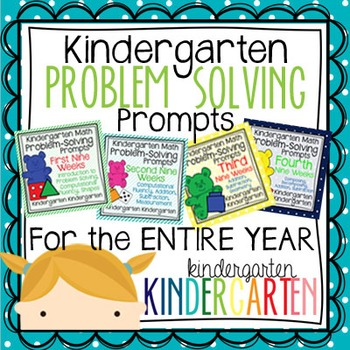Total:
\$0.00# Kindergarten Math Problem Solving Prompts for the Entire YearPreK - 1st
Subjects
Standards
Resource Type
Formats Included
• Zip
Pages
281 pages
\$20.00
List Price:
\$24.00
You Save:
\$4.00
\$20.00
List Price:
\$24.00
You Save:
\$4.00

### Description

This bundle includes all of my Kindergarten Problem-Solving Prompts for the entire year! That’s 180 engaging, rigorous and student-centered prompts that help students learn essential math skills, think critically, and communicate mathematical ideas in a meaningful way.
Here’s a preview of what you’ll get:
First 9 Weeks: Introduction to problem solving, how to record in math notebooks, how to share mathematical thinking, basic counting skills, comparing numbers, composing/decomposing numbers, introduction to addition/subtraction, 2-D shapes, position words.
Second Nine Weeks: Classifying and counting, a deeper exploration of addition/subtraction, counting to 20, comparing numbers, measurement (length, weight).
Third Nine Weeks: Addition/subtraction (learning how to write equations), 2-D and 3-D shapes.
Fourth Nine Weeks: Addition/subtraction, composing/decomposing numbers, making 10, teen numbers as “10 and some more”.
Each week includes teaching tips and notes for the teacher as well as questioning prompts to deepen students' mathematical understandings during share time.

Each problem has a cut and paste prompt. I have also included separate Microsoft Word files with editable 1 x 2.63” labels.

Included in this bundle:
Kindergarten Problem Solving Prompts FIRST Nine Weeks: CCSS Version
Kindergarten Problem Solving Prompts SECOND Nine Weeks: CCSS Version
Kindergarten Problem Solving Prompts THIRD Nine Weeks: CCSS Version
Kindergarten Problem Solving Prompts FOURTH Nine Weeks: CCSS Version

If you like these prompts, please don't forget to leave feedback!
Total Pages
281 pages
N/A
Teaching Duration
N/A
Report this Resource to TpT
Reported resources will be reviewed by our team. Report this resource to let us know if this resource violates TpT’s content guidelines.

### Standards

to see state-specific standards (only available in the US).
Count to 100 by ones and by tens.
Compare two numbers between 1 and 10 presented as written numerals.
Decompose numbers less than or equal to 10 into pairs in more than one way, e.g., by using objects or drawings, and record each decomposition by a drawing or equation (e.g., 5 = 2 + 3 and 5 = 4 + 1).
For any number from 1 to 9, find the number that makes 10 when added to the given number, e.g., by using objects or drawings, and record the answer with a drawing or equation.
Fluently add and subtract within 5.